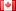# Revision history [back]

### matplotlib don't work??

import matplotlib.pyplot as plt

A = matrix([[1,2,3],[4,5,6],[7,8,9]])

plt.matshow(A)

What am I missing?

### matplotlib syntax don't work??

import matplotlib.pyplot as plt

A = matrix([[1,2,3],[4,5,6],[7,8,9]])

plt.matshow(A)

What am I missing?sage: matrix([2,3,4])

### syntax matplotlib don't work??

sage: matrix([2,3,4])import matplotlib.pyplot as plt

A = matrix([[1,2,3],[4,5,6],[7,8,9]])

plt.matshow(A)

What am I missing?

### matplotlib don't work??

import matplotlib.pyplot as plt

A = matrix([[1,2,3],[4,5,6],[7,8,9]])

plt.matshow(A)

What am I missing?

### matplotlib don't work??

What am I missing?6 restored questionDSM5242 ●21 ●68 ●112

### matplotlib don't work??

import matplotlib.pyplot as plt
A = matrix([[1,2,3],[4,5,6],[7,8,9]])
plt.matshow(A)


What am I missing?7 retaggedFrédéricC5011 ●3 ●42 ●109

### matplotlib don't work??

import matplotlib.pyplot as plt
A = matrix([[1,2,3],[4,5,6],[7,8,9]])
plt.matshow(A)


What am I missing?